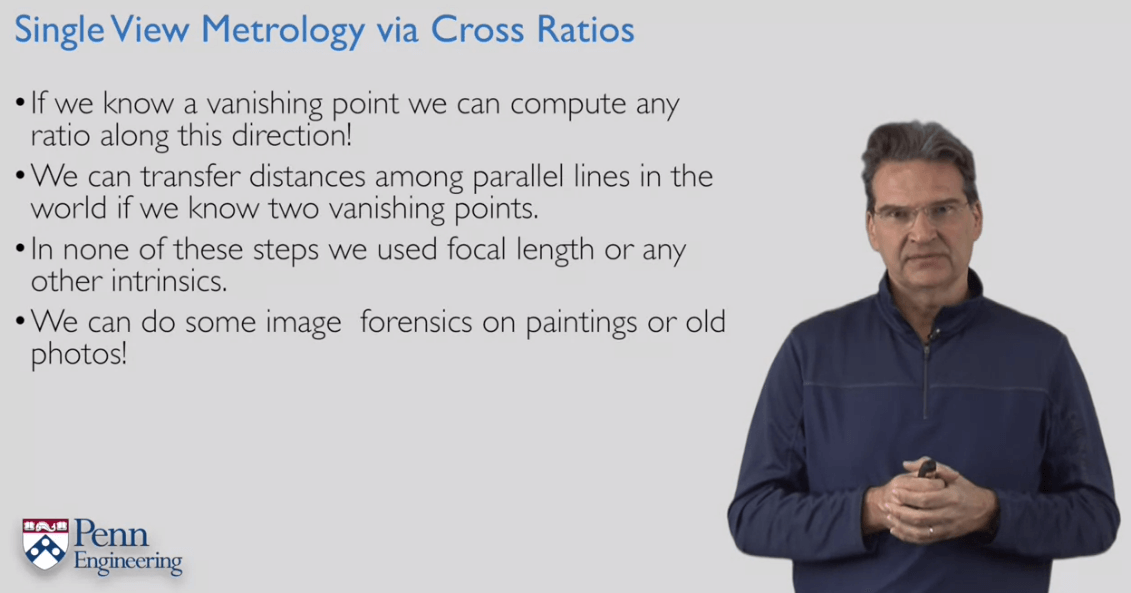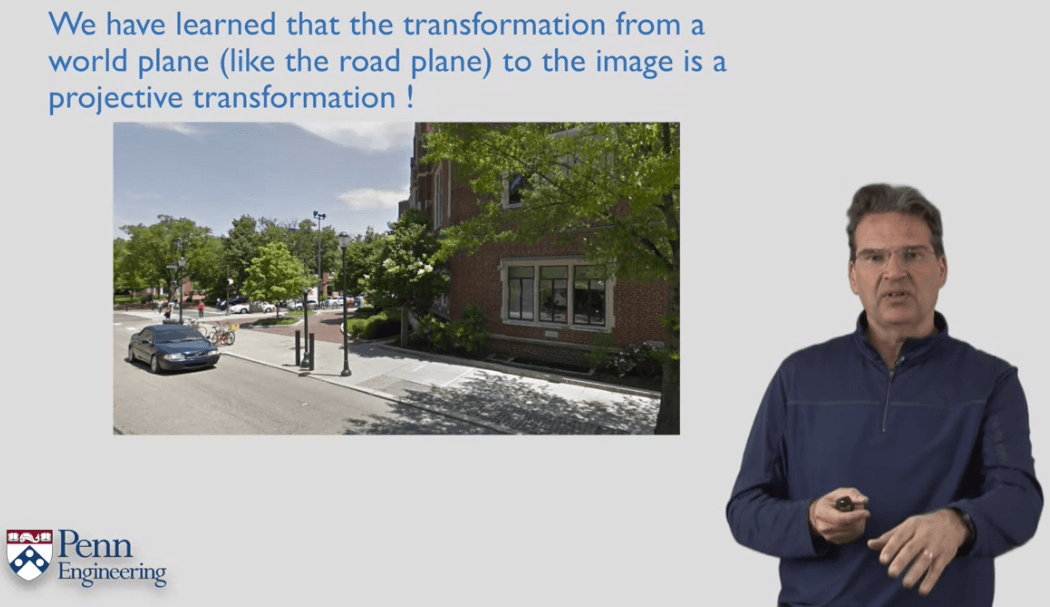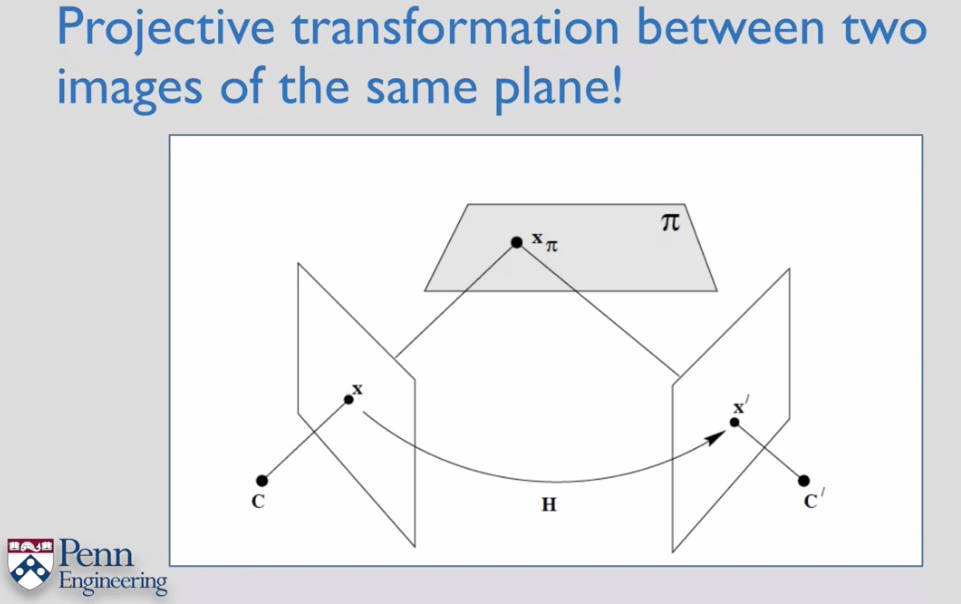# Robotics:Perception课程笔记2

## Oct 27,2019   4905 words   18 min

Tags: SLAM

#### 1.Vanishing Points-How to Compute Camera Orientation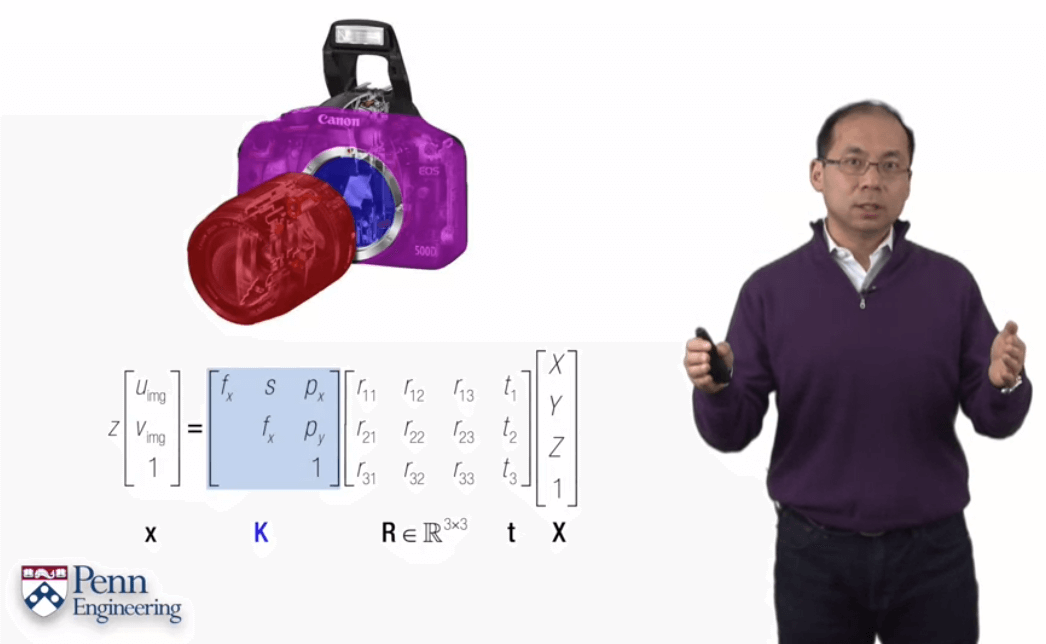将一个三维点转换到像素的过程有两个关键因素：一是相机拍摄时的R和t(外参)，二是相机的内参矩阵K。

##### 平面情况下的单应变换

###### 灭点计算相机姿态实例

$\begin{pmatrix} 533.18 & 0 & 342.22\\ 0 & 533.18 & 235.01\\ 0 & 0 & 1 \end{pmatrix}$

$\begin{matrix} l_{1}=(184,55,1)\times (132,452,1)=(-397,-52,75908)\\ l_{2}=(471,38,1)\times (520,449,1)=(-411,49,191719)\\ l_{3}=(184,55,1)\times (471,38,1)=(16,287,-18729)\\ l_{4}=(132,452,1)\times (520,449,1)=(3,388,-175772) \end{matrix}$

$\begin{matrix} v_{x}=l_{3}\times l_{4}=(16,287,-18729)\times (3,388,-175772)=(-43179712,2756165,5347)\\ v_{y}=l_{1}\times l_{2}=(-397,-52,75908)\times (-411,49,191719)=(-13688880,44914255,-40825) \end{matrix}$

$\begin{matrix} v_{x}=(-43179712,2756165,5347)/5347=(-8075.5,515.5,1)\\ v_{y}=(-13688880,44914255,-40825)/-40825=(335.3,-1100.2,1) \end{matrix}$

$\begin{matrix} r_{1}=K^{-1}z V_{x}\\ r_{2}=K^{-1}z V_{y} \end{matrix}$

$\begin{matrix} r_{1}=(-84417,2812,5347)\\ r_{2}=(530,102230,-40830) \end{matrix}$

$\begin{matrix} r_{1}=(-0.9974,0.0332,0.0632)\\ r_{2}=(0.0048,0.9287,-0.3709) \end{matrix}$

$r_{3}=r_{1}\times r_{2}=(-0.0710,-0.3696,-0.9265)$

$R = \begin{pmatrix} r_{1} & r_{2} & r_{3} \end{pmatrix}=\begin{pmatrix} -0.9974 & 0.0048 & -0.0710\\ 0.0332 & 0.9287 & -0.3696\\ 0.0632 & -0.3709 & -0.9265 \end{pmatrix}$

$\begin{matrix} \alpha = tan^{-1}(r_{3}(1)/r_{3}(3))=0.0765\\ \beta = sin^{-1}r_{3}(2)=-0.3786 \end{matrix}$

#### 2.Compute Projective Transformations

##### 投影变换的定义与性质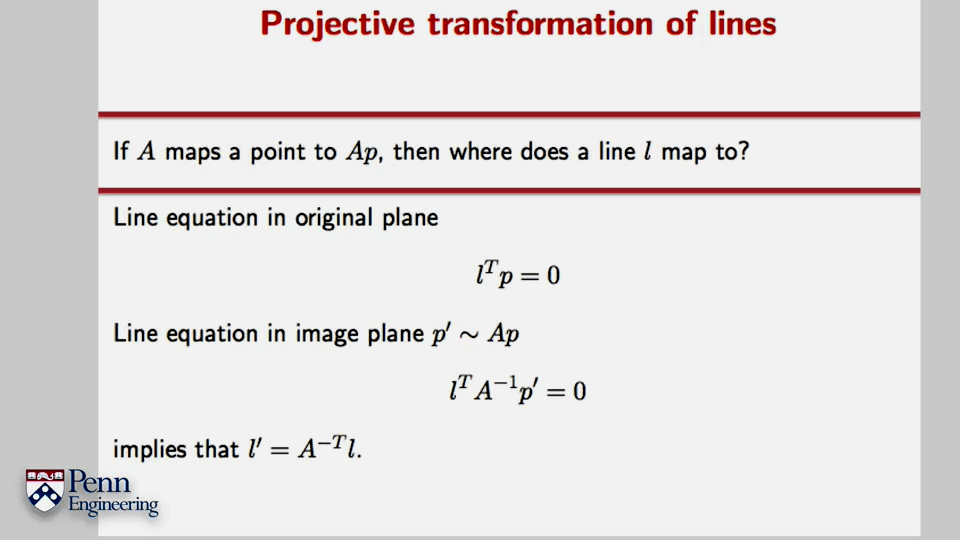直线在投影变换下的变换。设p’等于Ap，带回直线方程即可得到第二个式子，那么按照第一个式子直线方程的形式对应，就可以得到l’。这里l为直线方程的系数构成的向量，p为直线上的某点。

##### 投影变换矩阵的计算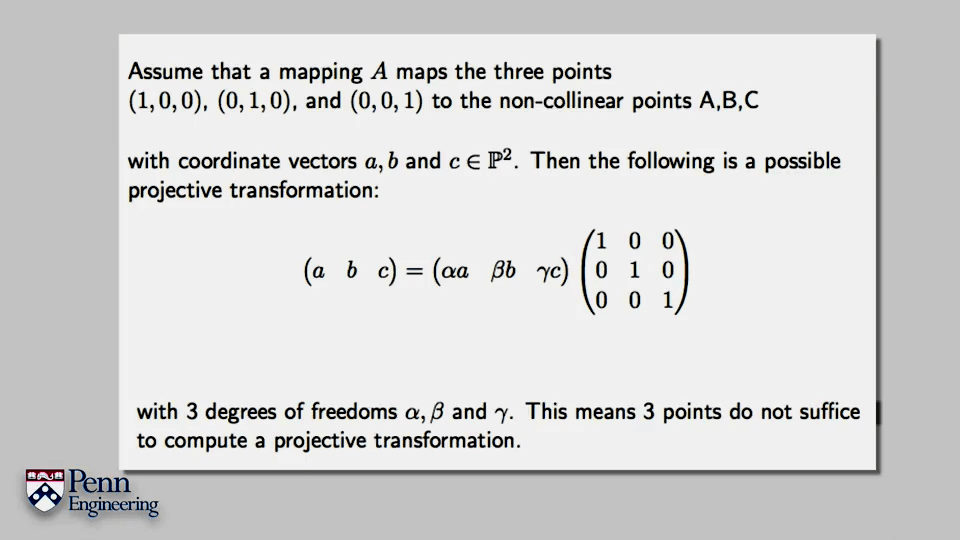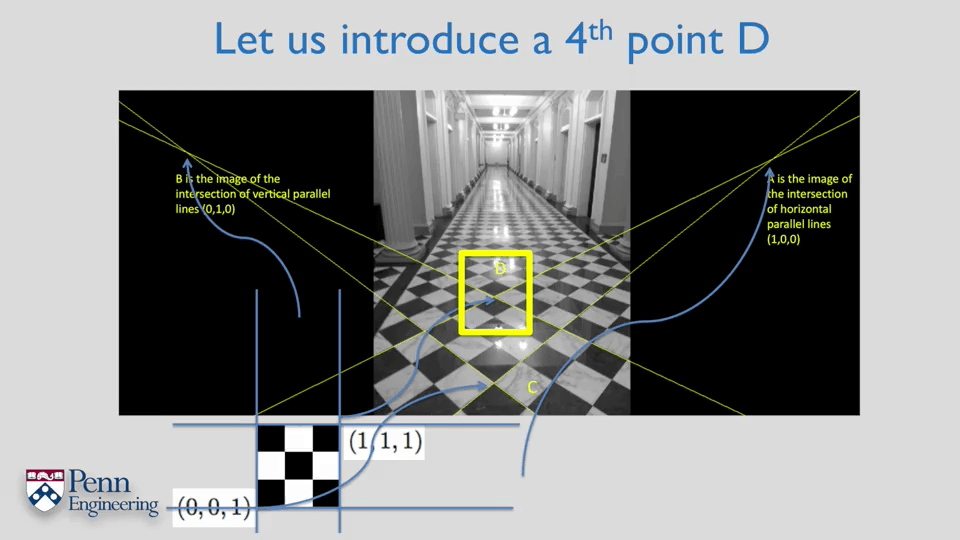至少需要4个对应点即可计算投影变换。简单来说就是利用三个非共线的点确定变换矩阵的“样子”(哪些元素非0，哪些元素是0)，再用最后一个点确定非零元素的值。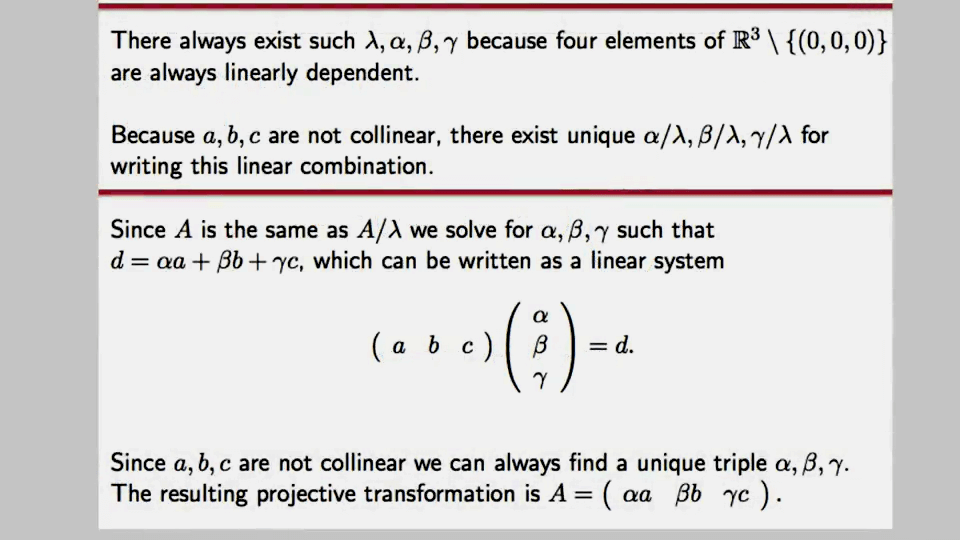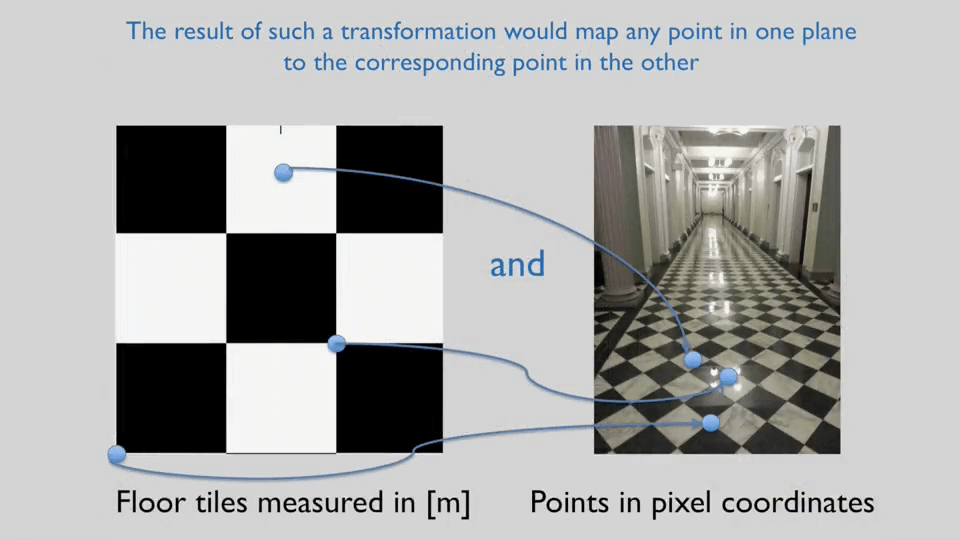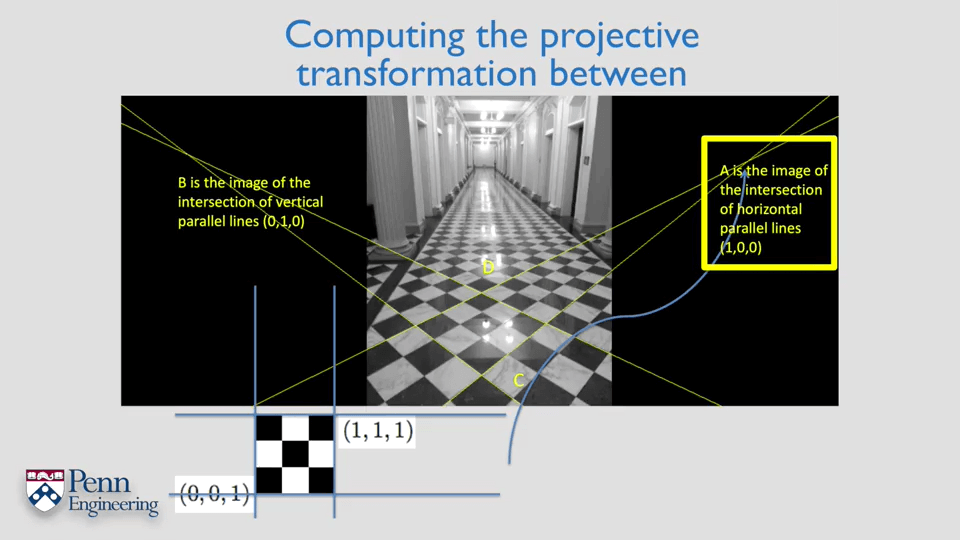对于两张影像上不知道真实坐标，只知道像素坐标的情况，如何计算两张影像间的投影变换呢？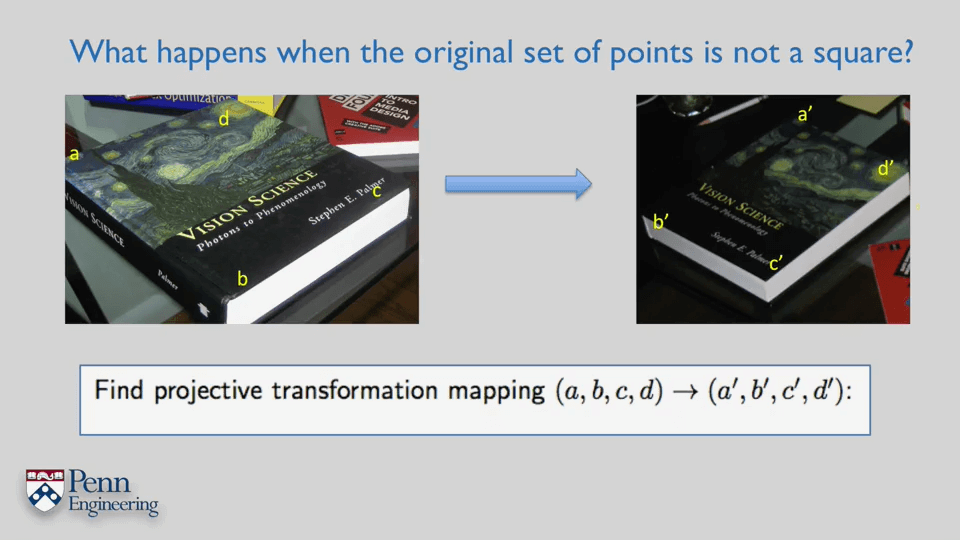简单来说就是构建虚拟坐标点，先求出虚拟点到第一张影像的投影变换，再求出虚拟点到第二张影像的投影变换，最后对两个运动代数方法合并两个变换，如下图中的第三个式子，即可得到两张影像间的变换了。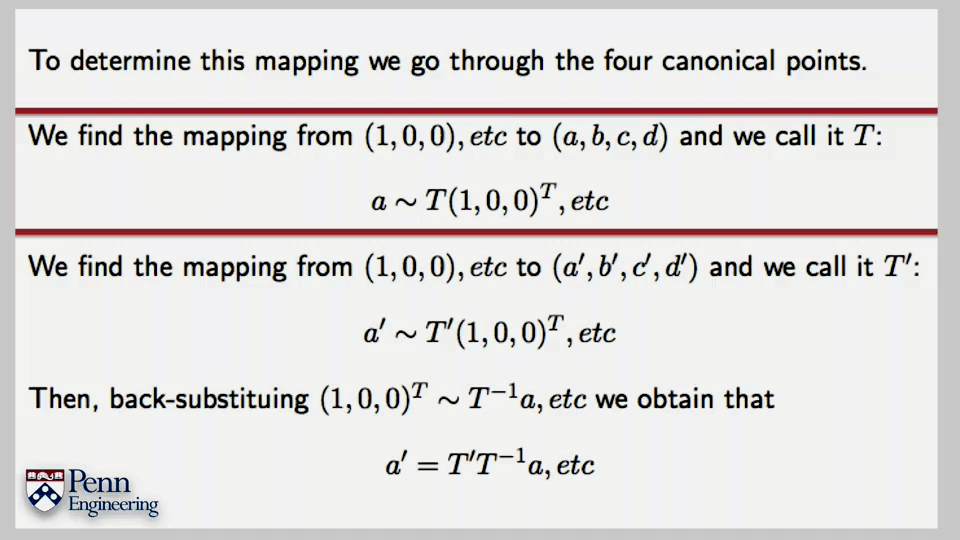#### 3.Projective Transformations and Vanishing Points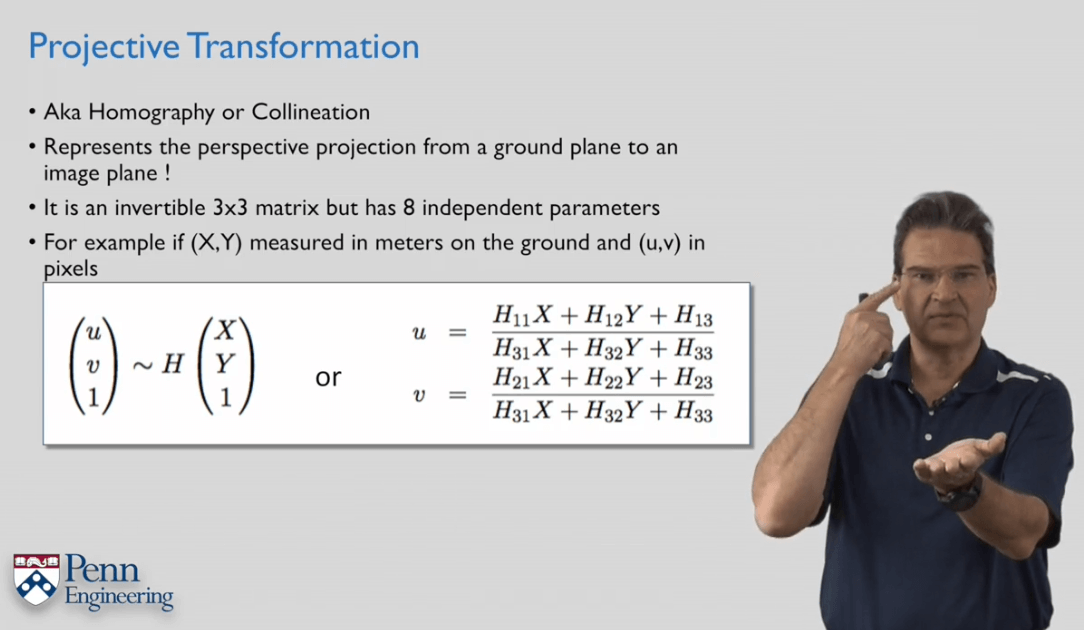一个例子：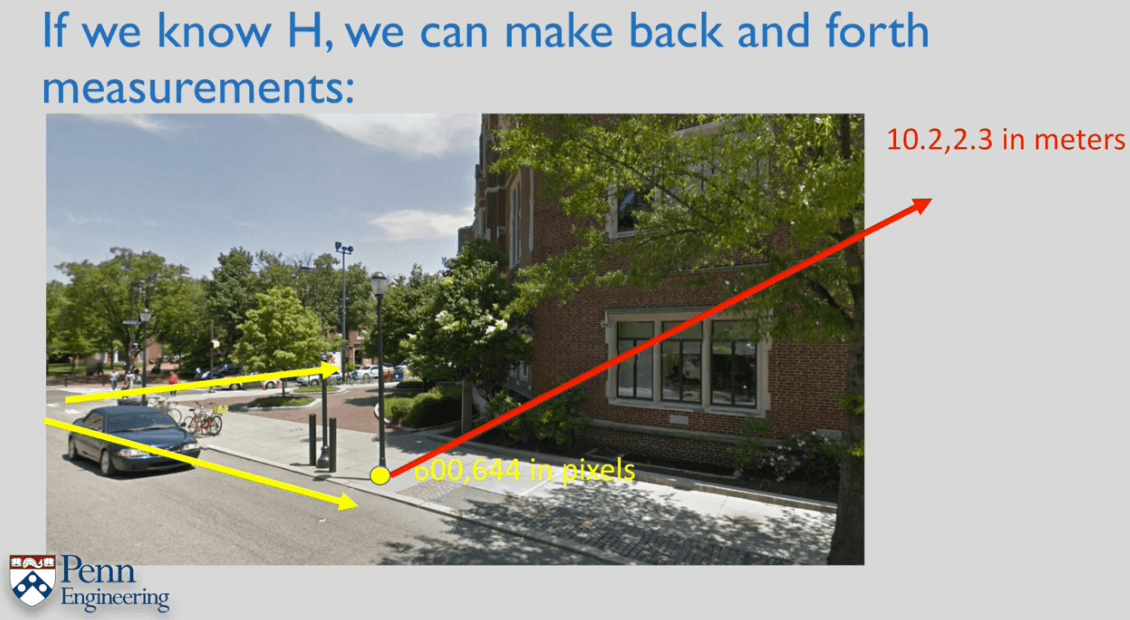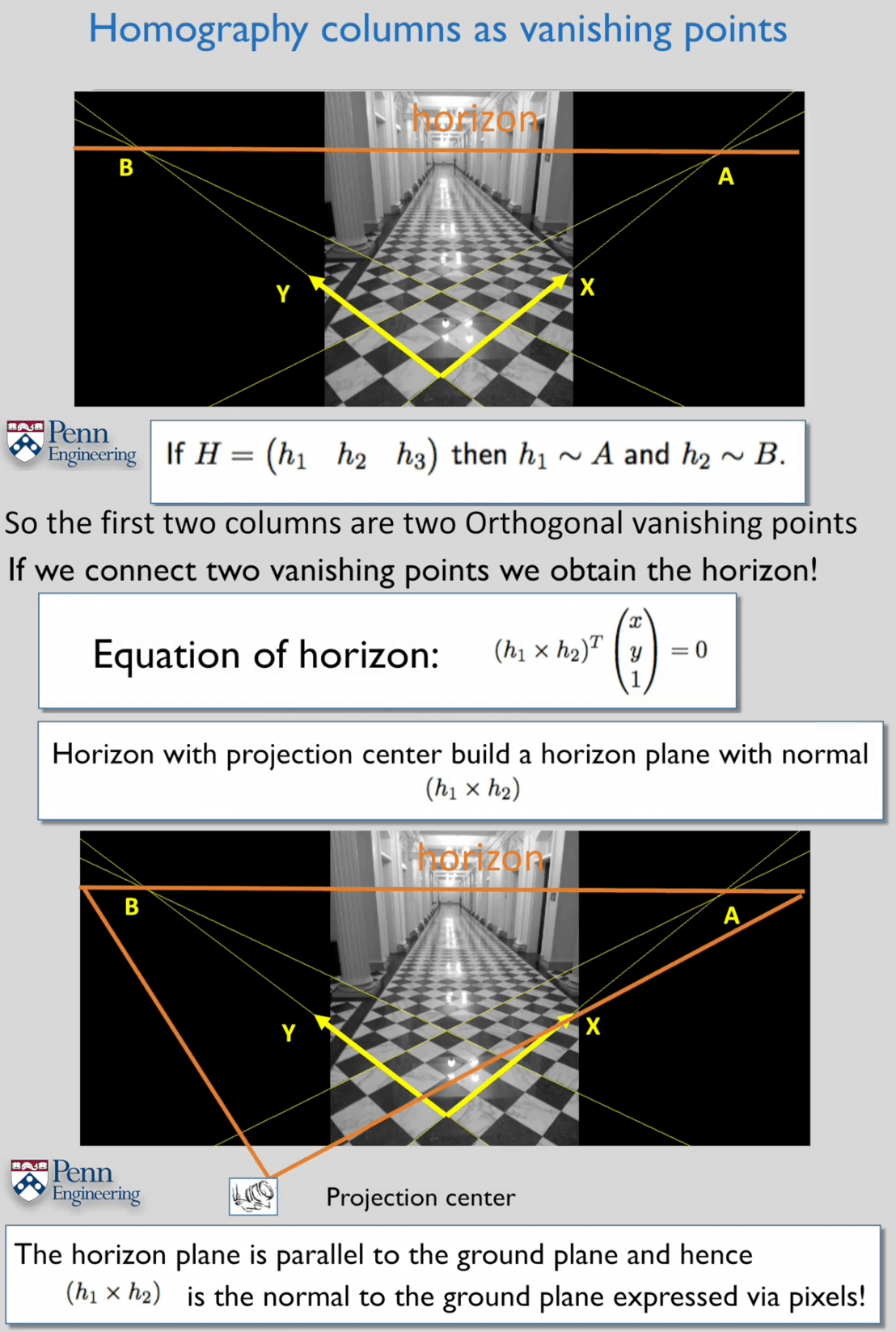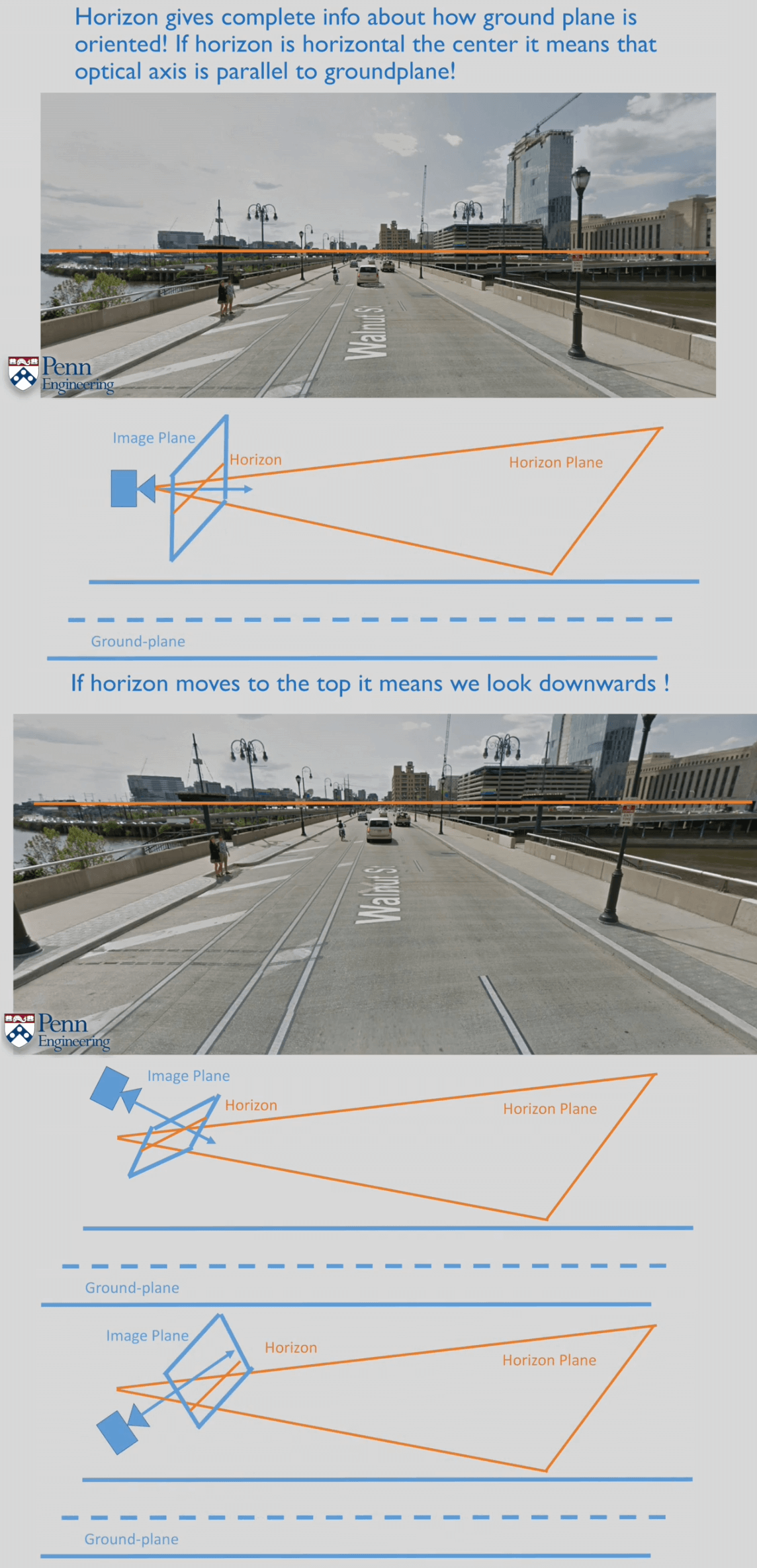#### 5.Two View Metrology